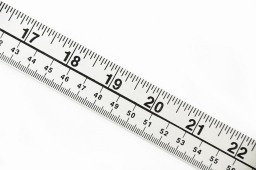# Centimeters 66264

The average height of the basketball team's primary five players is 196 cm. The underscore player is 216 cm tall. What is the average height of the remaining four players in centimeters?

x =  191 cm

### Step-by-step explanation:Did you find an error or inaccuracy? Feel free to write us. Thank you!

Tips for related online calculators
Looking for help with calculating arithmetic mean?
Looking for a statistical calculator?
Do you have a linear equation or system of equations and looking for its solution? Or do you have a quadratic equation?

#### You need to know the following knowledge to solve this word math problem:

We encourage you to watch this tutorial video on this math problem: